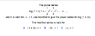# Interval of Convergence: Is it an Interval of Convergence Question?

• TyErd
Okay I understand how to do interval of convergences but how would I get the new series through substitution?You would use the substitution t = 1 - x to get the new series.f

## Homework Statement

I've attached the question

## The Attempt at a Solution

I'm not exactly sure how to do this question. Is it an interval of convergence question where i simply let log(1+2x) < 1 and solve for x??

#### Attachments

•aaaaaaaaaaaaaaaaaaaaaaa.png
3.7 KB · Views: 340
It's actually more of a logic problem. They told you log(1+x) converges for |x|<1. So what's the range of convergence of log(1+2x)? You are overthinking this by quite a bit.

## Homework Statement

I've attached the question

## The Attempt at a Solution

I'm not exactly sure how to do this question. Is it an interval of convergence question where i simply let log(1+2x) < 1 and solve for x??
No. What I would do is find the power series for log(1 + 2x), which is simply a matter of a substitution, and then determine the interval of convergence of the new series.

BTW, calculus-type problems like this should not be posted in the Precalc section.

No. What I would do is find the power series for log(1 + 2x), which is simply a matter of a substitution, and then determine the interval of convergence of the new series.

BTW, calculus-type problems like this should not be posted in the Precalc section.

I don't think it's even much of a precalc question. See my previous post.

I don't think it's even much of a precalc question. See my previous post.
I agree that it's not a difficult problem, but being about series and convergence, it seems a better place for it is in the Calculus & Beyond section.

I agree that it's not a difficult problem, but being about series and convergence, it seems a better place for it is in the Calculus & Beyond section.

Your choice, of course. But I don't think you need any convergence tests to answer the question. x is a number, 2x is a number.